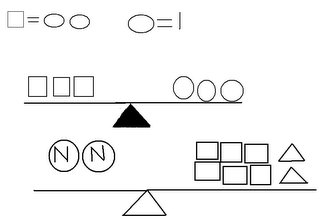## Sunday, May 28, 2006

### Janna's Growing Post for May 29th 2006

Question 1: What is the difference between an algerbraic expression and an equation (Hint: One contains this and the other doesn't)? Give an example of both an expression and an equation.

An algebraic expression is when you combine numbers, operatives, grouping symbols (such as brackets and parentheses) and/or free variables and bound variables. You get to choose the variable in an algebraic expression. An example of an algebraic expression is 20J / 5. In an algebraic equation is when you combine numbers, operatives, grouping symbols (such as brakets and parentheses) and/or free variables and bouund variables. In an equation the value of the variable is given. A example for an algebraic equation is 20J / 5 = 20. J equals 5.

Question 2: What is a variable and why do we use one in algebra?

A variable is a letter that replaces a number we are solving for. The variable stands for the unknown. We use a variable (a letter) in algebra so the equations we are solving do not become confusing. Example is we use numbers as variables instead of letters.
21 + 13 = 17 This equation doesn't make sense. Now here's the same equation except we use letters for the variable. 2A + 13 = 17. A eaquals 2. You multiply 2 by A which is 2. 2 x 2= 4. Your final equation would be 4 + 13 = 17.

Question 3: Solve for N.I found that since 2 circles equals 1 square and a circle equals one, that a square equals 2. To solve the second question you need to figure out what a triangle equals on the first scale. I took away four circles (each square equals two circles), and it left me with five circles. Since there were five circles left the triangle equals five circles each. Then I needed to solve the N's. If each square equals 2 and each triangle equals f circles each which is 10. I added it up and got 22. that means you now have to divide 22 in half and you'd get 11. So N eqauls 11.

Question 4: Solve the following equations. Show all of the steps that are needed.

a.) 3n+4n+7=2n+12
7n+7=2n+127n-2n(+7-7)=2n-2n(+12-7)
5n=5
N=1

b) 8n-(4+9)=1
1 8(3)-(4+9)=
1124-13=11
N= 3

(c) (8-3)n+7n+8=
4n+405n+7n+8=
4n+405(4)+7(4)+8=
4(4)+4020+28+8=56
N= 4

Question 5: For this question you need to create a T-Chart and a graph to plot your data from this question onto.

You are having a race against your friend, except you are on foot and he is on his bike. Your both know that if you are on a bike you will be faster so your friend gives you a head start of 3 minutes. If you can run 400m per minute, and your friend can bike 700m per minute, who will be the first to make it to the finish line 2000m away?The runner will cross the finish line first in 5 minutes. The biker will cross the finish line second in 6 minutes.

At 5/30/2006 9:23 AM,amelia_ mal said...

Hey Janna! that was great! i think you deserve full marks. Amlia

At 5/30/2006 3:34 PM,Fang_the_Barbarian said...

GREAT JOB!!!!

At 6/01/2006 3:57 PM,Mr. Reece said...

Janna where is question 5?

As well you should be solving to find the value of n in question 4, not substituting a value for n and then solving it 4b.

For question 1, what is given in a equation that isn't in an expression that allows us to find the value of the variable?

otherwise it seems great.

At 6/19/2006 2:35 PM,Mr. Reece said...

Janna you did not post a T-chart and graph for question 5. Your mark is 24/30

At 1/30/2018 11:23 PM,Love Kpop said...

I'm puzzled with lots of exercises. I was afraid I could not do the right time despite my hard work. I need a support person.
http://run3play.com